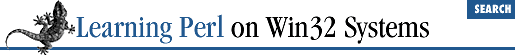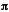home | O'Reilly's CD bookshelfs | FreeBSD | Linux | Cisco | Cisco Exam

#This appendix gives the answers for the exercises found at the end of each chapter.

## A.1 Chapter 2, Scalar Data

1. Here's one way to do it:

```\$pi = 3.141592654;
\$result = 2 * \$pi * 12.5;
print "radius 12.5 is circumference \$result\n";```

First, we give a constant value () to the scalar variable ``` \$pi``` . Next, we compute the circumference using this value of ``` \$pi``` in an expression. Finally, we print the result using a string containing a reference to the result.

2. Here's one way to do it:

```print "What is the radius: ";

\$pi = 3.141592654;
\$result = 2 * \$pi * \$radius;

This is similar to the previous exercise, but in this case, we've asked the person running the program for a value, using a ``` print``` statement for a prompt, and then the ``` <STDIN>``` operator to read a line from the terminal.

If we had left off the ``` chomp``` , we'd get a newline in the middle of the displayed string at the end. You must get that newline off the string as soon as you can.

3. Here's one way to do it:

```print "First number: "; chomp(\$a = <STDIN>);
print "Second number: "; chomp(\$b = <STDIN>);
\$c = \$a * \$b; print "Answer is \$c\n";```

The first line does three things: prompts you with a message, reads a line from standard input, and then gets rid of the inevitable newline at the end of the string. Note that because we are using the value of ``` \$a``` strictly as a number, we can omit the ``` chomp``` here, because ``` 45\n``` is 45 when used numerically. However, such careless programming would likely come back to haunt us later on (for example, if we were to include ``` \$a``` in a message).

The second line does the same thing for the second number and places it into the scalar variable ``` \$b``` .

The third line multiplies the two numbers together and prints the result. Note the newline at the end of the string here, contrasted with its absence in the first two lines. The first two messages are prompts, for which user input was desired on the same line.

4. Here's one way to do it:

```print "String: "; \$a = <STDIN>;
print "Number of times: "; chomp(\$b = <STDIN>);
\$c = \$a x \$b; print "The result is:\n\$c";```

As with the previous exercise, the first two lines ask for, and accept, values for the two variables. Unlike the previous exercise, we don't ``` chomp``` the newline from the end of the string, because we need it! The third line takes the two entered values and performs a string repetition on them, and then displays the answer. Note that the interpolation of ``` \$c``` is not followed by a newline, because we believe that ``` \$c``` will always end in a newline anyway.19.6 ExercisesA.2 Chapter 3, Arrays and List Data